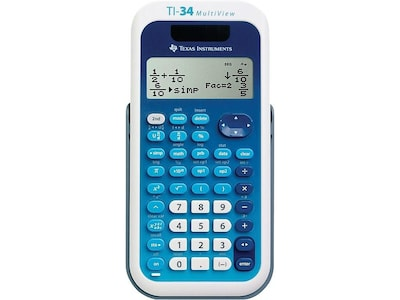Can you use an extra \$850 annually? Become a member today.
Close

# Texas Instruments MultiView TI-34 16 Digit Scientific Calculator, Blue/White

Item #: 901-733108TEX
Model #: TI-34 MULTIVIEW
Brand: Texas Instruments\$22.99 Each
Delivery Date:

Enter Zip Code
Description
Improve mathematical understanding and solve problems quickly with this Texas Instruments TI-34 MultiView scientific calculator. Enhance student engagement and boost test scores with this Texas Instruments TI-35 MultiView scientific calculator. Ideal for students, this advanced calculator lets users compare the results of their calculations and helps develop pattern recognition skills with an easy-to-read four-line LCD display. This calculator helps deepen understanding of mathematical symbols and notation with the built-in MathPrint function, which shows students how numbers and expressions appear in textbooks. The list conversion function helps students internalize number formats and conversions by displaying formats side-by-side, ideal for improving fundamental math skills. This Texas Instruments TI-35 MultiView scientific calculator helps students learn about fractions with built-in integer division and step-by-step fraction simplification functions.
• Scientific calculator with 16-digit four-line LCD display
• Perfect choice for middle school math, pre-algebra, algebra 1 and 2, general science, geometry
• Calculator works from solar cell and one (CR2032) battery
• Provides up to seven memory variables
• One- and two-variable statistics with stat variable input storage, EOS (Equation Operating System), up to eight pending operations, up to 23 levels of parentheses, error recovery capability
• MathPrint mode for input in math notation, including p, square roots, fractions, percents and exponents plus math notation output for fractions
• Calculator provides: fraction calculations, fraction/decimal conversions, hyperbolic functions, trigonometry, log and antilog functions
• 1-year manufacturer limited warranty
Specifications
 Brand : Texas Instruments Color Family : White Customizable : No Features : One- and two-variable statistics,Advanced fraction capabilities Number of Digits Displayed : 16 Number of Lines : 4 Power Source : Battery/Solar Solar Powered : Yes True Color : Blue and White Used For : General math, Algebra, Geometry, Weight : 0.52 lbs. per Each Weight : 0.52 lbs. per Each

### Customers Also ViewedLoading...